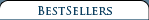3-View Scale Airplane Drawing Books & CDs Aero Albums Books Air Force Legends Air Racing Books Airliner Books Aviation CDs Aviation Century Series Biographicals British Aviation Books Children's Aviation Books Civil Aviation Books A-G Civil Aviation Books H-N Civil Aviation Books O-S Civil Aviation Books T-Z DVDs & Videos Early Aviation Books Golden Age Era Golden Age Of Aviation Series Military Aviation Modeling & R/C Airplane Books Naval Fighters Navy Books Nose Art Books Paul Matt Individual 3-View Drawings Personal Adventures Post World War II Books Soaring, Ultralights & Ballooning Time-Life's Epic Of Flight Series Type Airplane Books A-G Type Airplane Books H-P Type Airplane Books R-Z World War I Aviation Books World War II Aviation Books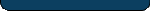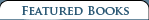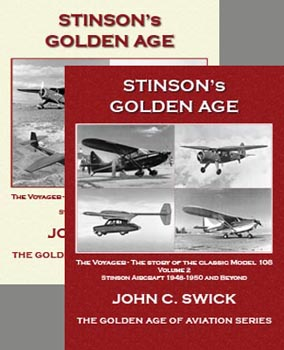Stinson's Golden Age - Vol 1 & Vol 2 \$ 50.00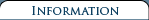Shipping and Returns   Terms and Conditions  Privacy Policy  Contact Us### Bellanca\\\\\\\'s Golden Age

 Retail Price: \$24.25 By: Alan Abel and Drina Welch Abel Bellanca's Golden Age is included in The Golden Age of Aviation Series. Bellanca's Golden Age book examines the colorful history of Giuseppe Mario Bellanca, better known as G.M. Bellanca, and his company's aircraft. This book's rich narrative and numerous period photographs reveal the reasons why the young Sicilian moved to the U.S. in 1910, and includes Bellanca innovations such as Columbia, which flew non-stop from New York to Germany just two weeks after Lindbergh's flight; Miss Veedol, the aircraft that made the first non-stop transpacific flight; the Cruisemaster; Bellanca racers and others. This is the only book that captures the complete Bellanca story. Details of the first 10 flights over the Atlantic and the first one over the Pacific are provided. Includes never-before-told stories of Charles Lindbergh, Clarence Chamberlin and several other Golden Age personalities.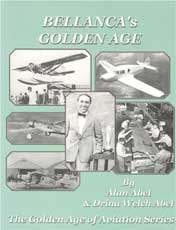Tells the complete story of the greatest airplane of the 1920s--Bellanca's Columbia, the plane Lindbergh wanted for his famous flight, but did not get. Lindbergh thought an agreement for purchase had been reached, but Lindbergh left angry and immediately put into place plans for the Spirit of St. Louis.

In the 1930s the government tested 209 airplanes and found that Bellanca's was the best regarding "efficiency."

Book details Bellanca airplanes including Bellanca CD, CE, CF, Wright-Bellanca 1, WB-2, Columbia, Bellanca J, TES Tandem, Pacemaker, Special J-300, Model K, Airbus/Aircruiser, C-27A, Miss Veedol, The White Falcon, Senior Skyrocker, The Flash, XSE-1, XSE-2, Bellanca Racer, Cruisair Junior, YO-50 (liaison) AT-21-BL Trainer, Cruisair Senior, and Cruisemaster.

The Golden Age of Aviation Series highlights a number of aircraft manufacturers during the 1900s, 1910s, 1920s, 1930s & 1940s. The series presents an historical look at AviationвЂ™s Golden Years through detailed text, numerous photos, internationally acclaimed 3-view airplane scale drawings, archival materials and original magazine ads of the era.

BOOK REVIEWS for The Golden Age of Aviation Series:

Smithsonian's Air & Space: "Archival photos and technical drawings abound."

American Aviation Historical Society: "...details the people, facilities and manufacturing operations."

EAA's Sport Aviation: "...progressively tells the events and details of these aircraft."

Skyways: "a jewelвЂ¦. extraordinaryвЂ¦. marvelousвЂ¦. 3-views augment excellent photos and text.вЂќ

General Aviation News: "...books ARE A MUST FOR AVIATION BUFFS."

Historic Aviation: вЂњвЂ¦.with rich narrative and colorful histories.вЂќ

BellancaвЂ™s Golden Age
Book Contents

ChapterВ В В В В В В В В В В В В В В В В В В В В В В В В В В В В В В В В В В В В В В В В В В В В В В В В В В В В В В В В В В В В В В В В В В В В В В В  Page

1В В В В В В В В В  BellancaвЂ™s YouthВ В В В В В В В В В В В В В В В В В В В В В В В В В В В В В В В В В В В В В В В В В В В В В В В В В В В В В В В В В В В В В В В В В В В В  1
2В В В В В В В В В  Coming to AmericaВ В В В В В В В В В В В В В В В В В В В В В В В В В В В В В В В В В В В В В В В В В В В В В В В В В В В В В В В В В В В В В В В В В  2
3В В В В В В В В В  Maryland Pressed Steel CompanyВ В В В В В В В В В В В В В В В В В В В В В В В В В В В В В В В В В В В В В В В В В В  4
4В В В В В В В В В  The 1919 Model CE 55-hp BiplaneВ В В В В В В В В В В В В В В В В В В В В В В В В В В В В В В В В В В В В В В В В  7
5В В В В В В В В В  The Roos-Bellanca Airplane Company & the Model CFВ В В В В В В В В В  10
6В В В В В В В В В  A Taste of IndependenceВ В В В В В В В В В В В В В В В В В В В В В В В В В В В В В В В В В В В В В В В В В В В В В В В В В В В В В В В В  14
7В В В В В В В В В  The Wright Aeronautical CorporationВ В В В В В В В В В В В В В В В В В В В В В В В В В В  15
8В В В В В В В В В  The Wright-Bellanca Number 1 (WB-1)В В В В В В В В В В В В В В В В В В В В В В В В В В В В В В В В В В  16
9В В В В В В В В В  The Wright ApacheВ В В В В В В В В В В В В В В В В В В В В В В В В В В В В В В В В В В В В В В В В В В В В В В В В В В В В В В В В В В В В В В В В  18
10В В В В В В В  The WB-2В В В В В В В В В В В В В В В В В В В В В В В В В В В В В В В В В В В В В В В В В В В В В В В В В В В В В В В В В В В В В В В В В В В В В В В В В В В В В В В  20
11В В В В В В В  The Columbia & The Endurance RecordВ В В В В В В В В В В В В В В В В В В В В В В В В В В В В В В В В В  22
12В В В В В В В  Charles Lindbergh, the Columbia & ParisВ В В В В В В В В В В В В В В В В В В В В В В В В В В В В В В В В  24
13В В В В В В В  Air Transportation Over Great DistancesВ В В В В В В В В В В В В В В В В В В В В В В В В В В В В В В В В В  28
14В В В В В В В  Chamberlin & Levine Make Record FlightВ В В В В В В В В В В В В В В В В В В В В В В В В В В В В В В  35
15В В В В В В В  The Man of the Hour, The Toast of the TownВ В В В В В В В В В В В В В В  38
16В В В В В В В  A Parting of the WaysВ В В В В В В В В В В В В В В В В В В В В В В В В В В В В В В В В В В В В В В В В В В В В В В В В В В В В В В В В В В В В В  40
17В В В В В В В  The Bellanca JВ В В В В В В В В В В В В В В В В В В В В В В В В В В В В В В В В В В В В В В В В В В В В В В В В В В В В В В В В В В В В В В В В В В В В В В В В  42
18В В В В В В В  The Bellanca Tandem Experimental SesquiplaneВ В В В В В В В В В В В В В В В В В В В В В В  44
19В В В В В В В  The Bellanca PacemakerВ В В В В В В В В В В В В В В В В В В В В В В В В В В В В В В В В В В В В В В В В В В В В В В В В В В В В В В В В В  47
20В В В В В В В  The Bellanca Special J-300В В В В В В В В В В В В В В В В В В В В В В В В В В В В В В В В В В В В В В В В В В В В В В В В В В В В В В  55В В В В В В В В В В В В В В В В В В В В В В В В В В В В В В В
21В В В В В В В  5,000 Miles in a Flying Gas TankВ В В В В В В В В В В В В В В В В В В В В В В В В В В В В В В В В В В В В В В В В В В В В  57
22В В В В В В В  The Bellanca Model KВ В В В В В В В В В В В В В В В В В В В В В В В В В В В В В В В В В В В В В В В В В В В В В В В В  61
23В В В В В В В  The Flying WВ В В В В В В В В В В В В В В В В В В В В В В В В В В В В В В В В В В В В В В В В В В В В В В В В В В  В В В В В В В В В В В В В В В В В В В В В В В  64
24В В В В В В В  More Record-Setting FlightsВ В В В В В В В В В В В В В В В В В В В В В В В В В В В В В В В В В В В В В В В В В В В В В В В В В В В  70
25В В В В В В В  More 1930s Bellanca вЂ“ Another Grand DecadeВ В В В В В В В В В В В В В В В В В В В В В В  76В В В В В В В В В В В В В В В В В В В
26В В В В В В В  The Bellanca Senior SkyrocketВ В В В В В В В В В В В В В В В В В В В В В В В В В В В В В В В В В В В  79
27В В В В В В В  The Bellanca FlashВ В В В В В В В В В В В В В В В В В В В В В В В В В В В В В В В В В В В В В В В В В В В В В В В В В В В В В В В В В В В В В В В В В В  81
28В В В В В В В  The Bellanca XSE-1 & XSE-2В В В В В В В В В В В В В В В В В В В В В В В В В В В В В В В В В В В В  85
29В В В В В В В  Bellanca Builds a RacerВ В В В В В В В В В В В В В В В В В В В В В В В В В В В В В В В В В В В В В В В В В В В В В В В В В В В В В В В В В В  86
30В В В В В В В  BellancaвЂ™s First Venture into LightplanesВ В В В В В В В В В В В В В В В В В В В В В В В В В В В В В В В В В  87
31В В В В В В В  The Bellanca YO-50В В В В В В В В В В В В В В В В В В В В В В В В В В В В В В В В В В В В В В В В В В В В В В В В В В В В В В В В В В В В В В В  89
32В В В В В В В  World War II EffortВ В В В В В В В В В В В В В В В В В В В В В В В В В В В В В В В В В В В В В В В В В В В В В В В В В В В В В В В В В В В В В В В В  91
33В В В В В В В  Postwar BellancaВ В В В В В В В В В В В В В В В В В В В В В В В В В В В В В В В В В В В В В В В В В В В В В В В В В В В В В В В В В В В В В В В В В В В В  96
34В В В В В В В  BellancaвЂ™s Crowning Achievement, The Cruisair Senior 103
35В В В В В В В  The Bellanca CruisemasterВ В В В В В В В В В В В В В В В В В В В В В В В В В В В В В В В В В В В В В В В В В В В В В В В В В В В В В В  107
36В В В В В В В  After 42 Years вЂ¦ The End of the Bellanca EraВ В В В В В В В В В В В  111
37В В В В В В В  Into the New Era, The Business ClosesВ В В В В В В В В В В В В В В В В В В В В В В В  113
38В В В В В В В  G.M. BellancaвЂ™s DeathВ В В В В В В В В В В В В В В В В В В В В В В В В В В В В В В В В В В В В В В В В В В В В В В В  115
39В В В В В В В  Like Father, Like Son вЂ“ August BellancaВ В В В В В В В В В В В В В В В В В В В В В В В В В В В В В В В В В  118
40В В В В В В В  The Other Bellanca, 1956 to PresentВ В В В В В В В В В В В В В В В В В В В В В В В В В В В В В В В В В В В В В В В  121

Bellanca's Golden Age book includes 148 pgs, SB, 8.5 x 11, 175+ photos, 3-view outlines.

### Bellanca\\\\\\\'s Golden Age

#### Item Number: 82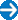Type in the title of book or similar words to help find your item(s).